# A Math Core Topics – Trigonometry and Further Trigo

## 12 Nov A Math Core Topics – Trigonometry and Further Trigo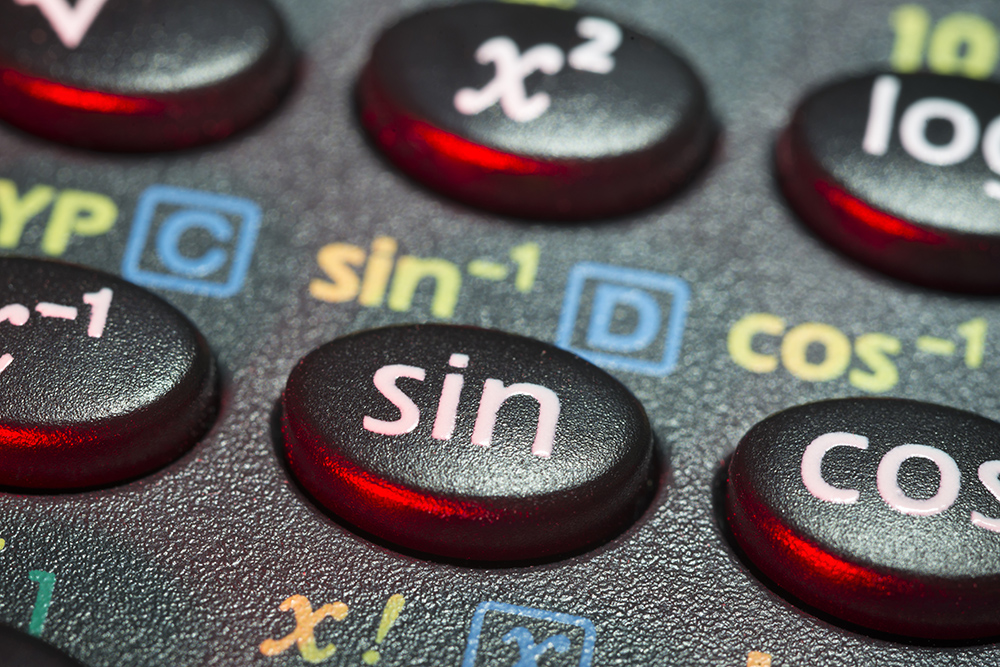#### Why trigonometry and further trigo are so important in A math?

Trigonometry is the study of the relationship between the angles and the sides of geometric figures. In the A math syllabus, students are required to study not only the fundamentals of trigonometry but also the more progressive use of trigo-related identities and formulae to manipulate equations to determine the values of angles and sides. Moreover, students have to learn the trigonometric graphs in A math to understand how the periodic function works with respect to the angles and the R formula, which allows students to calculate maximum or minimum lengths with their associated angles. Moreover, mastering trigonometry and further trigo will equip students with the necessary knowledge and skills for advanced mathematics in their further studies. In addition, trigonometry and further trigonometry will allow students to nurture and strengthen their reasoning skill, critical thinking skill, and analytic skills to connect ideas between mathematics and other subjects, like engineering, physics, etc.

#### What skills do the students need to have in order to master the topic?

Students are required to possess strong analytic skill, reasoning skill and critical thinking skill to connect the important concepts and apply them to solve the questions.

Students are frequently tested to prove the trigonometric identities. They need to remember the fundamental formulas of trigonometry which are not found in the formula sheet (for example, cos θ = 1sec θ ); they also need to be familiar with using the given formulas in the formula sheet to prove trigo identities.

The second type of questions which often appear in the exam is finding the maximum and minimum value of the sides of a given geometric figure and their respective angles. It is important for the students to be able to recall the R formula correctly in the exam since it is also not given in the formula sheet. Students need to have clear understanding about the sine/cosine graph and their respective maximum minimum values to be able to find the max/min sides. Knowledge of finding the effective ranges, basic angles are required to solve such questions so that the students will be able to reject those angles which are out of the given range.

Thirdly, the students have to be proficient in finding the angles based on the positive and negative sign of different trigonometric functions in different quadrants. Although many students are able to memorise the signs of different trigonometric functions in the respective quadrants, they must be able to apply them correctly in the question.

Very often, the students are tested on their understanding and application of the trigo concepts and methods as shown in the following example: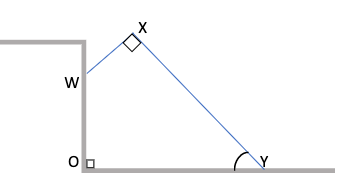The diagram shows an L shaped digger WXY resting against a vertical wall. WX= 3 m, XY= 12 m, ∠WXY=∠WOY=90° and ∠XYO=θ , 0°≤ θ ≤90°

1. Show that OY= (3 sin θ + 12 cos θ)m
2. Express OY in the form R cos(θ-α)
3. Find the maximum value of OY and the corresponding value of θ.
4. Find the value of θ for which OY = 9 m.

Solution: Draw the line XB parallel to vertical wall and line WA parallel to OY. Hence ∠WAX = 90°. The diagram will look like the following: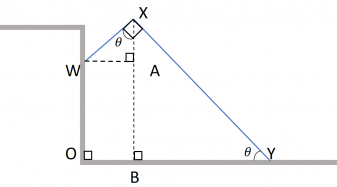In BXY, ∠BXY= 180°-90°- θ (Angle sum of )
∠BXY= 90°- θ
Hence, ∠WXA = 90°- ∠BXY = θ (adjacent angles)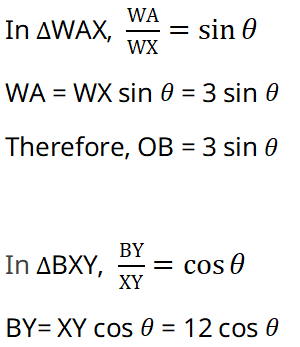(i) OY = OB + BY
= 3 sin θ+ 12 cos θ(Shown)

(ii) Comment: The students have to recall the R-formulas and know how to find the value of R and respectively.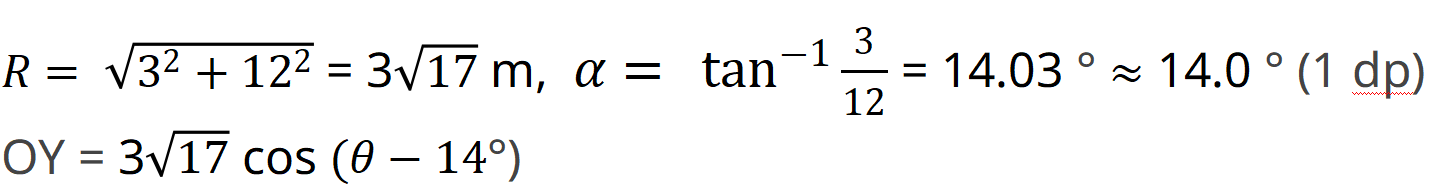(iii) according to the cosine graph,

#### How to score well in Trigonometry and Further Trigo in O level A math?

Both trigo and further trigo are the major topics in A Math which will resurface in other major topics such as Differentiation and Integration.  These topics will pull up the students’ grade in A Math if they are proficient in it.  Consistency in understanding and practices will definitely raise the students’ mastery and performance in both trigo and further trigo. This not only increases the students’ exposure of questions, but it will also sharpen their problem solving and application skillsTake regular tests on these topics with time limit to train the students up in time-management skills and application skills.  These help to prepare the students thoroughly for the upcoming WAs and exams in school and ultimately score well in A Math in the O level.

#### Einstein Takeaway

Both trigo and further trigo topics are content-heavy in the A Math syllabus.  For a systematic learning experience on A Math that enhances the understanding and accuracy of the applications via a step-by-step approach, contact Einstein for the free trial lesson.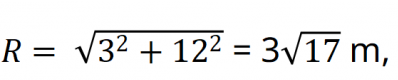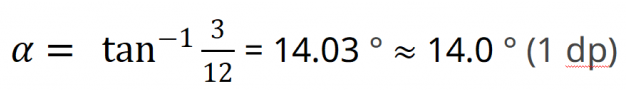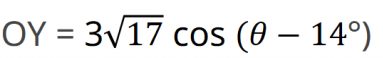(iii) according to the cosine graph,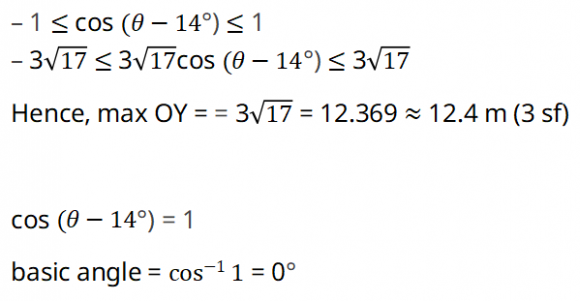Effective Range Calculation: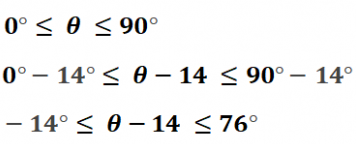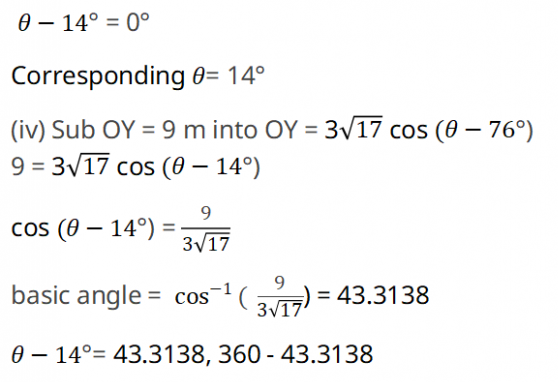= 43.3138, 316.6861 (rejected because θ
is less than 90° as shown in the diagram)
θ = 43.3138 + 14
θ = 57.5138 ≈ 57.5° (1 dp)

#### How to score well in Trigonometry and Further Trigo in O level A math?

Both trigo and further trigo are the major topics in A Math which will resurface in other major topics such as Differentiation and Integration.  These topics will pull up the students’ grade in A Math if they are proficient in it.  Consistency in understanding and practices will definitely raise the students’ mastery and performance in both trigo and further trigo. This not only increases the students’ exposure of questions, but it will also sharpen their problem solving and application skillsTake regular tests on these topics with time limit to train the students up in time-management skills and application skills.  These help to prepare the students thoroughly for the upcoming WAs and exams in school and ultimately score well in A Math in the O level.

#### Einstein Takeaway

Both trigo and further trigo topics are content-heavy in the A Math syllabus.  For a systematic learning experience on A Math that enhances the understanding and accuracy of the applications via a step-by-step approach, contact Einstein for the free trial lesson.